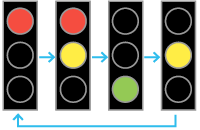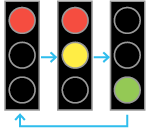Deals Of The Week - hours only!Up to 80% off on all courses and bundles.-Close
Recap
Tuples as return types in functions
Tuples as records
9. Tuples representing states or positions – traffic lights
List of tuples
Summary

## Instruction

Perfect! We can also create a tuple which describes the state of traffic lights:

# (red, yellow, green), True = light on
initial_state = (True, False, False)

The example above represents a red traffic light. Now, let's say the traffic lights follow the cycle shown below:We can write a function which, given a tuple with the traffic lights' current state, returns the next state:

def next_lights_state(current_state):
red, yellow, green = current_state
if red:
if yellow:
return (False, False, True)
else:
return (True, True, False)
else:
if yellow:
return (True, False, False)
else:
return (False, True, False)

The function above checks which lights are on at the moment and returns the next traffic light setting.

## Exercise

Write a similar function named next_simple_lights_state(current_state) that returns the next stage in the cycle shown below:Represent the light state as a tuple, the same way as shown in the explanation.

### Stuck? Here's a hint!

Unpack the current_state argument into three variables as shown in the explanation. Then, use the following template:

if red:
if yellow:
return...
else:
return...
else:
return...